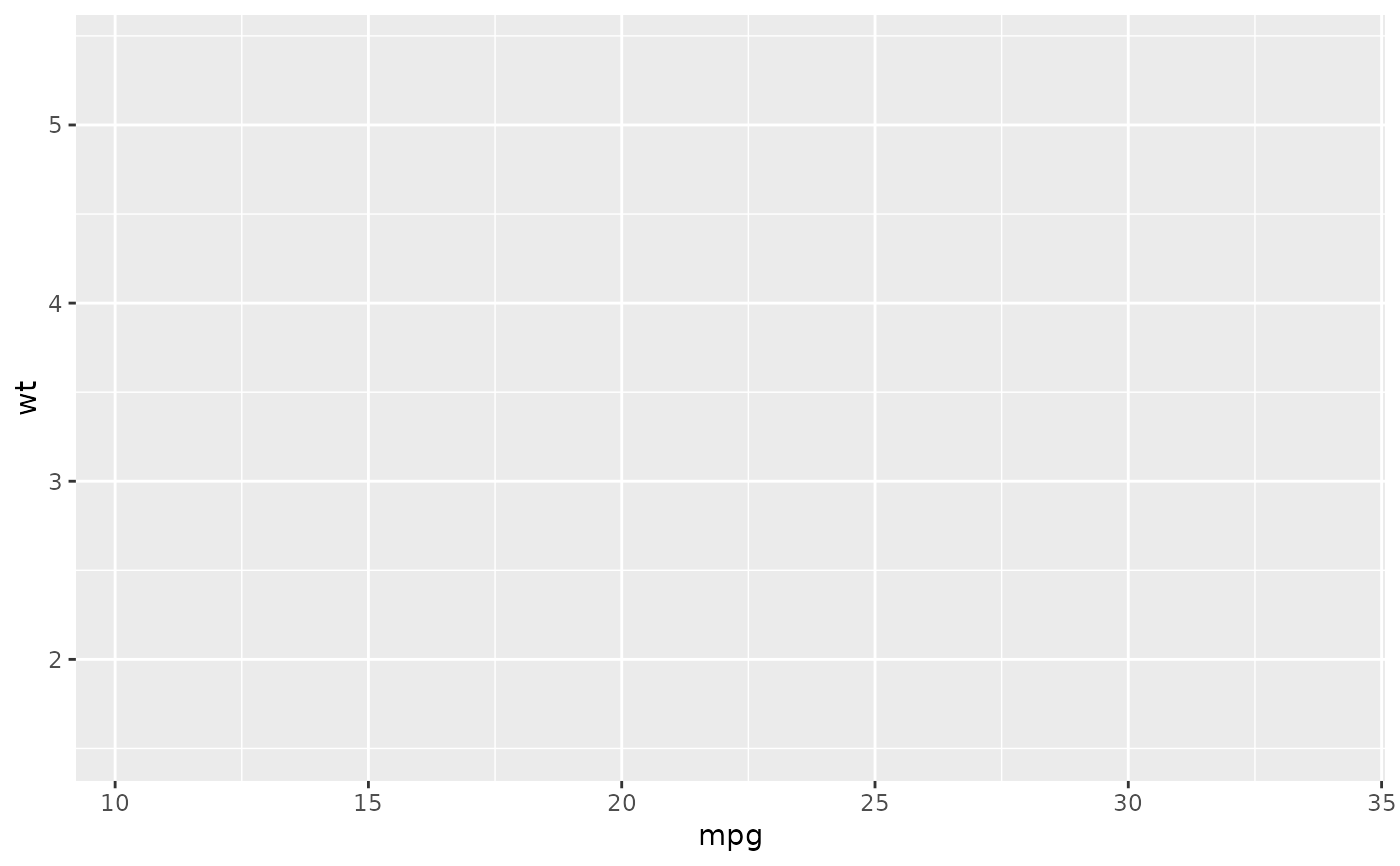Generate code to create a ggplot

ggcall(data = NULL, mapping = NULL, geom = NULL,
geom_args = list(), scales = NULL, scales_args = list(),
coord = NULL, labs = list(), theme = NULL, theme_args = list(),
facet = NULL, facet_args = list())

## Arguments

data Character. Name of the data.frame. List. Named list of aesthetics. Character. Name of the geom to use (without "geom_"). List. Arguments to use in the geom. Character vector. Scale(s) to use (without "scale_"). List. Arguments to use in scale(s), if scales is length > 1, must be a named list with scales names. Character. Coordinates to use (without "coord_"). List. Named list of labels to use for title, subtitle, x & y axis, legends. Character. Name of the theme to use (without "theme_"). List. Named list for theme arguments. Character vector. Names of variables to use as facet. List. Named list for facet arguments.

a call

## Examples

# Default:
ggcall()#> ggplot()
# With data and aes
ggcall("mtcars", list(x = "mpg", y = "wt"))#> ggplot(mtcars) + aes(x = mpg, y = wt)
# Evaluate the call
library(ggplot2)
eval(ggcall("mtcars", list(x = "mpg", y = "wt")))# With a geom:
ggcall(
data = "mtcars",
mapping = list(x = "mpg", y = "wt"),
geom = "point"
)#> ggplot(mtcars) + aes(x = mpg, y = wt) + geom_point()
# With options
ggcall(
data = "mtcars",
mapping = list(x = "hp", y = "cyl", fill = "color"),
geom = "bar",
coord = "flip",
labs = list(title = "My title"),
theme = "minimal",
facet = c("gear", "carb"),
theme_args = list(legend.position = "bottom")
)#> ggplot(mtcars) + aes(x = hp, y = cyl, fill = color) + geom_bar() +
#>     labs(title = "My title") + coord_flip() + theme_minimal() +
#>     theme(legend.position = "bottom") + facet_wrap(vars(gear,
#>     carb))
# One scale
ggcall(
data = "mtcars",
mapping = list(x = "mpg", y = "wt", color = "qsec"),
geom = "point",
scales = "color_distiller",
scales_args = list(palette = "Blues")
)#> ggplot(mtcars) + aes(x = mpg, y = wt, color = qsec) + geom_point() +
#>     scale_color_distiller(palette = "Blues")
# Two scales
ggcall(
data = "mtcars",
mapping = list(x = "mpg", y = "wt", color = "qsec", size = "qsec"),
geom = "point",
scales = c("color_distiller", "size_continuous"),
scales_args = list(
color_distiller = list(palette = "Greens"),
size_continuous = list(range = c(1, 20))
)
)#> ggplot(mtcars) + aes(x = mpg, y = wt, color = qsec, size = qsec) +
#>     geom_point() + scale_color_distiller(palette = "Greens") +
#>     scale_size_continuous(range = c(1, 20))